# RD Sharma Solutions for Class 10 Maths Chapter 10 Circles Exercise 10.2

This exercise has interesting and challenging questions based on the concept of a tangent to a circle, tangent from a point on a circle and length of a tangent. Our expert team at BYJU’S has created the RD Sharma Solutions Class 10 for students to help them clear conceptual doubts and to perform well in their exams. The right ways to solve questions of this exercise is important for students to understand and its available as RD Sharma Solutions for Class 10 Maths Chapter 10 Circles Exercise 10.2 PDF in the link provided below.

## RD Sharma Solutions for Class 10 Maths Chapter 10 Circles Exercise 10.2 Download PDF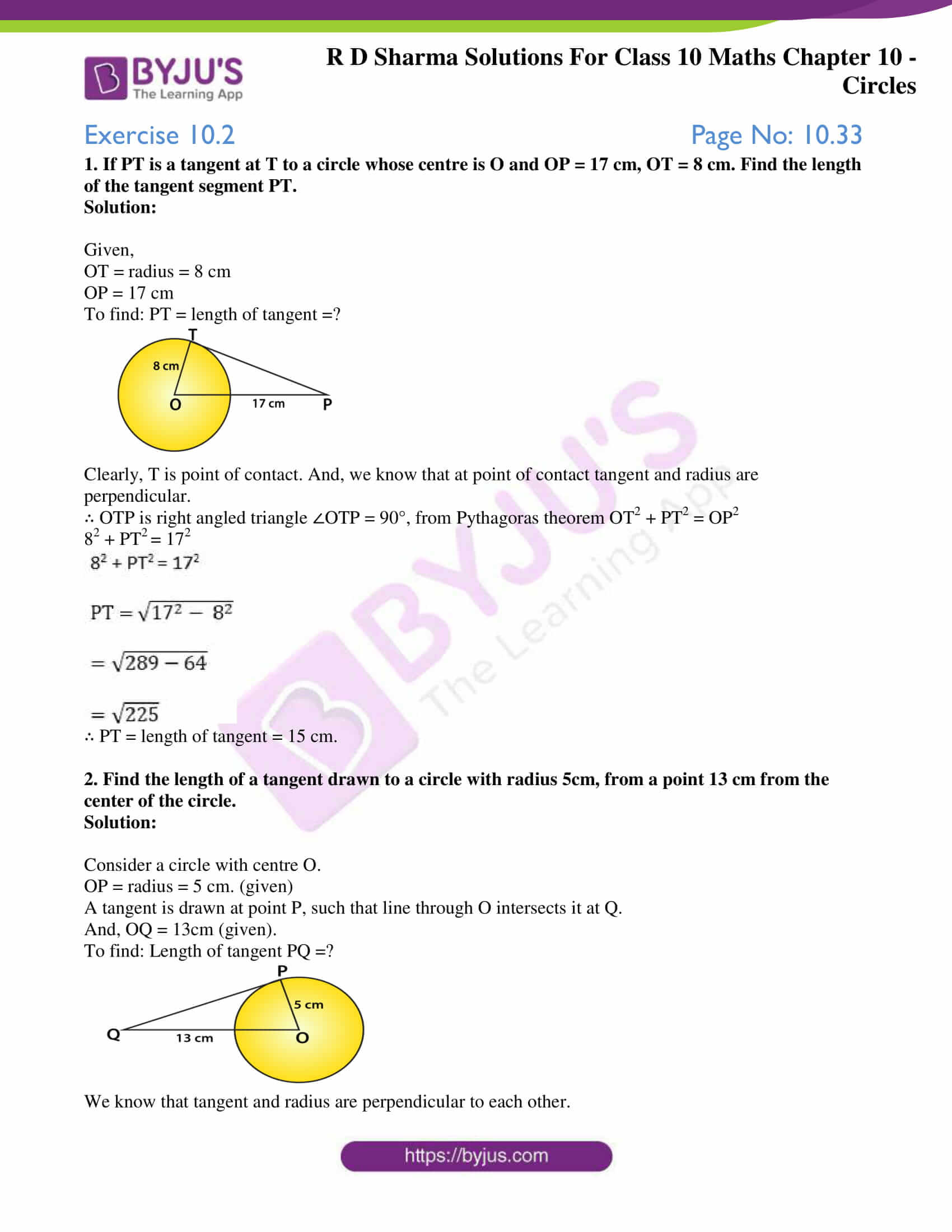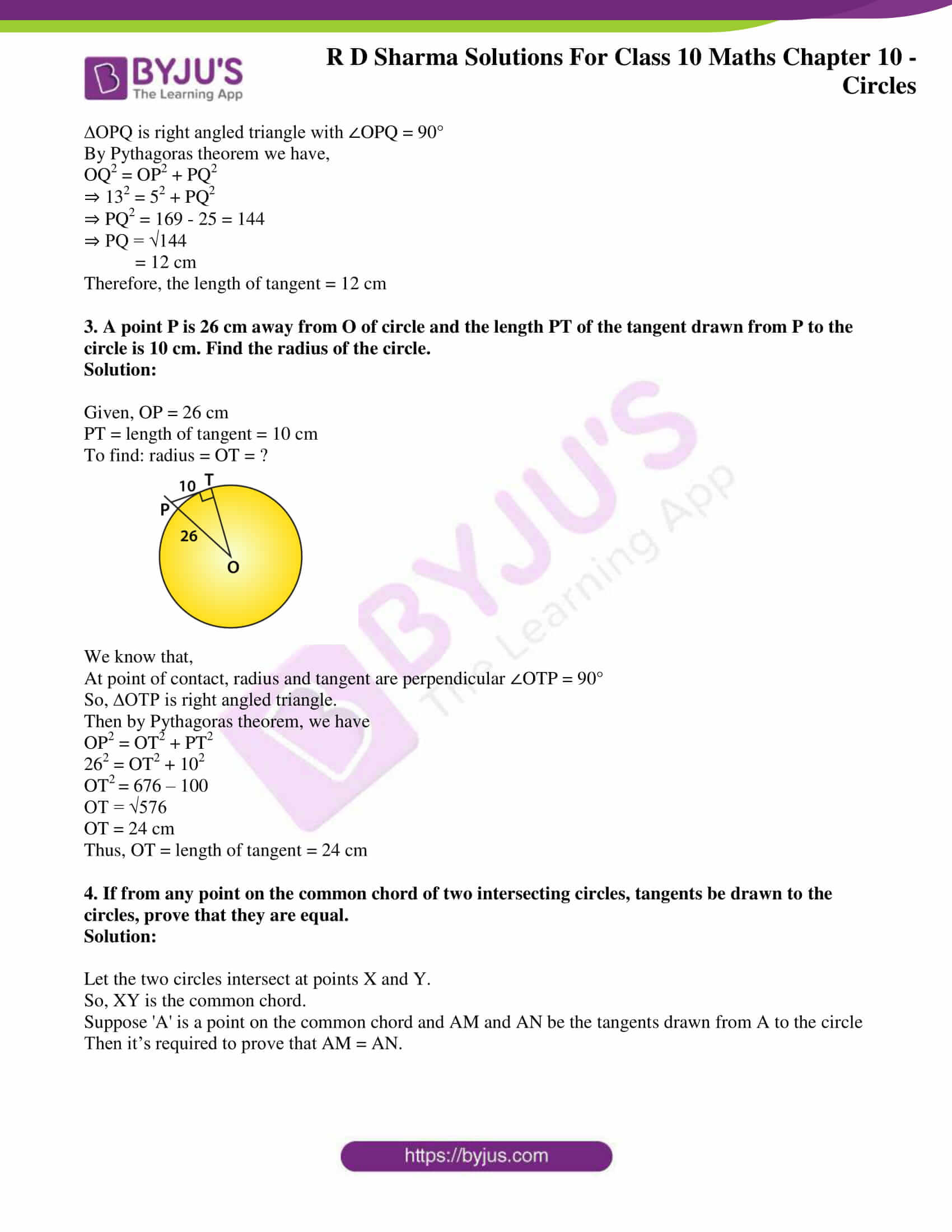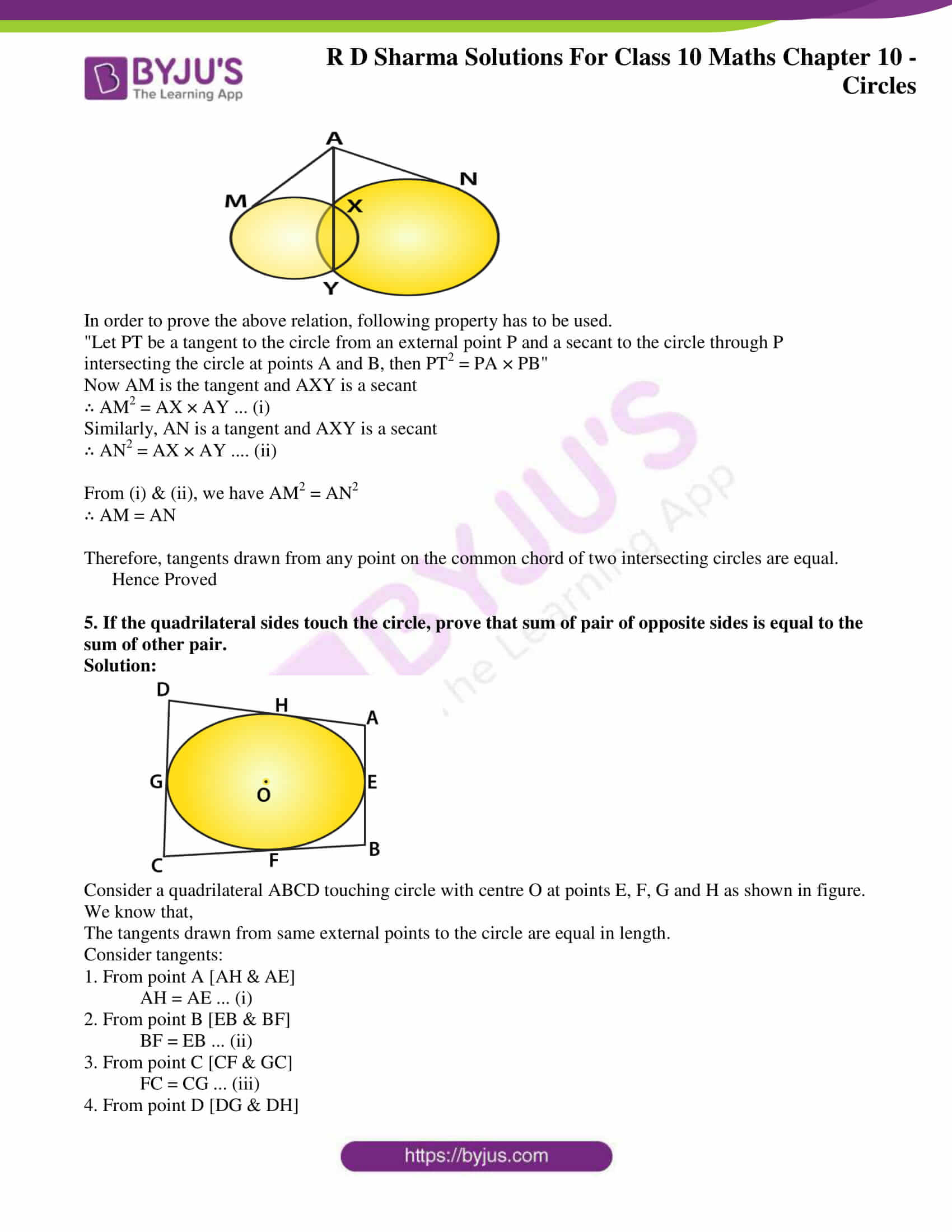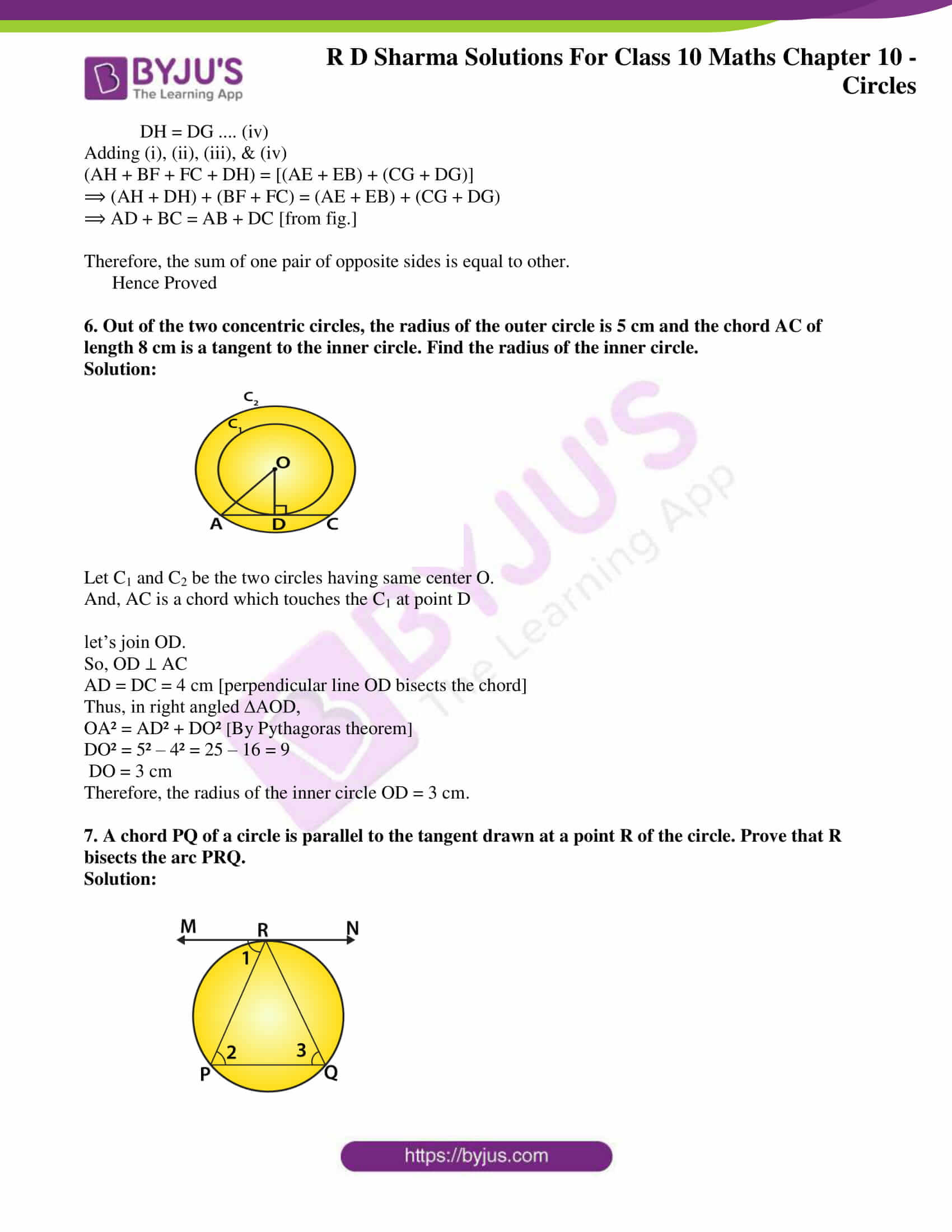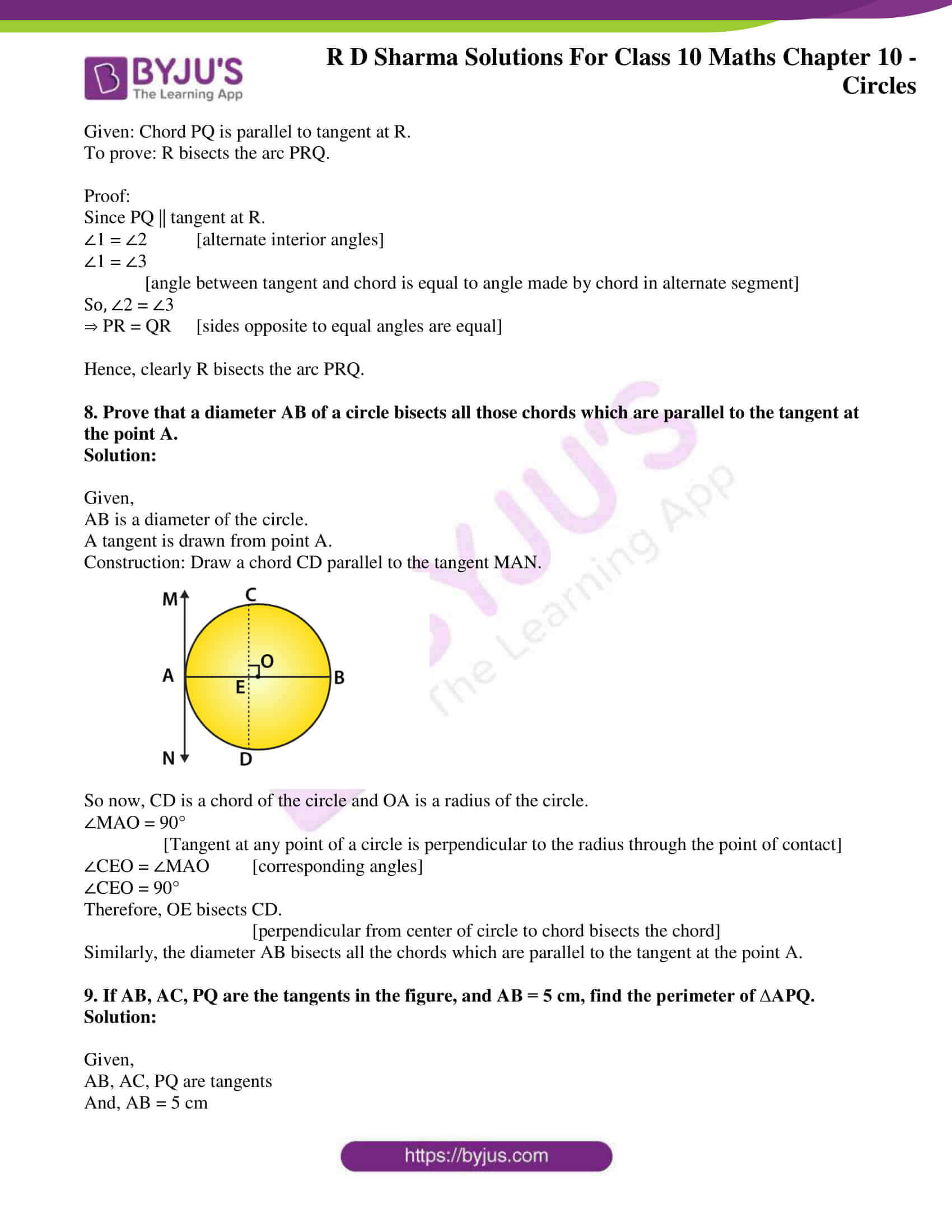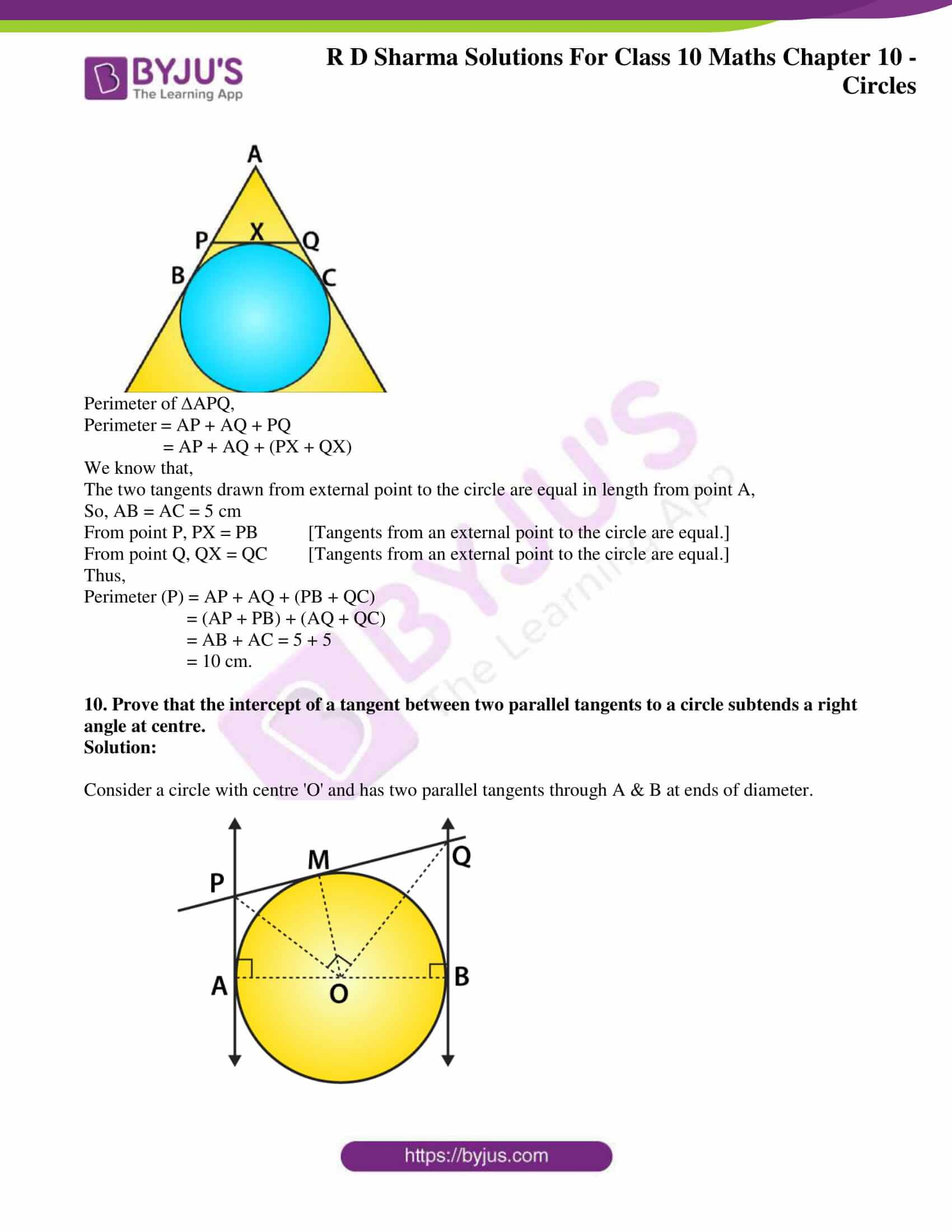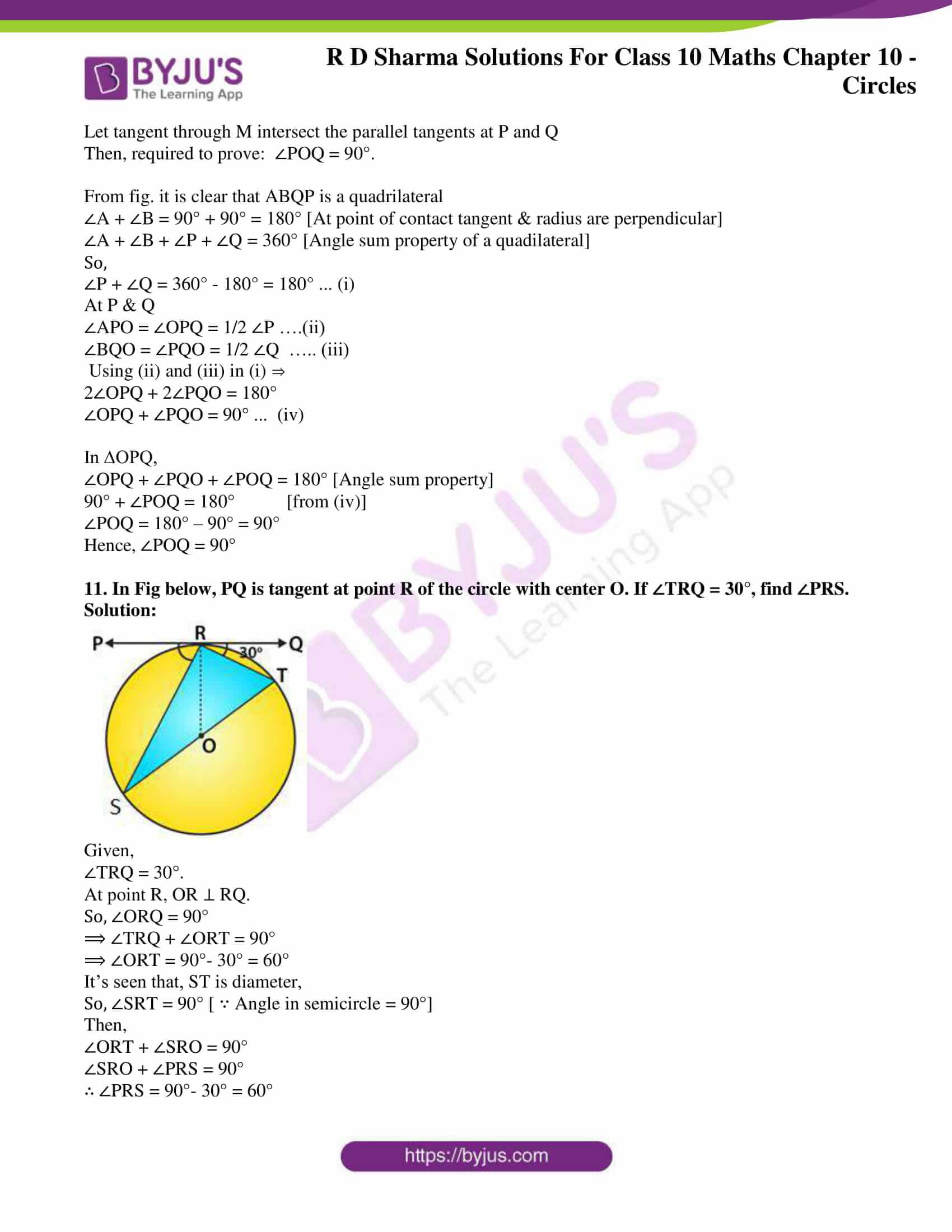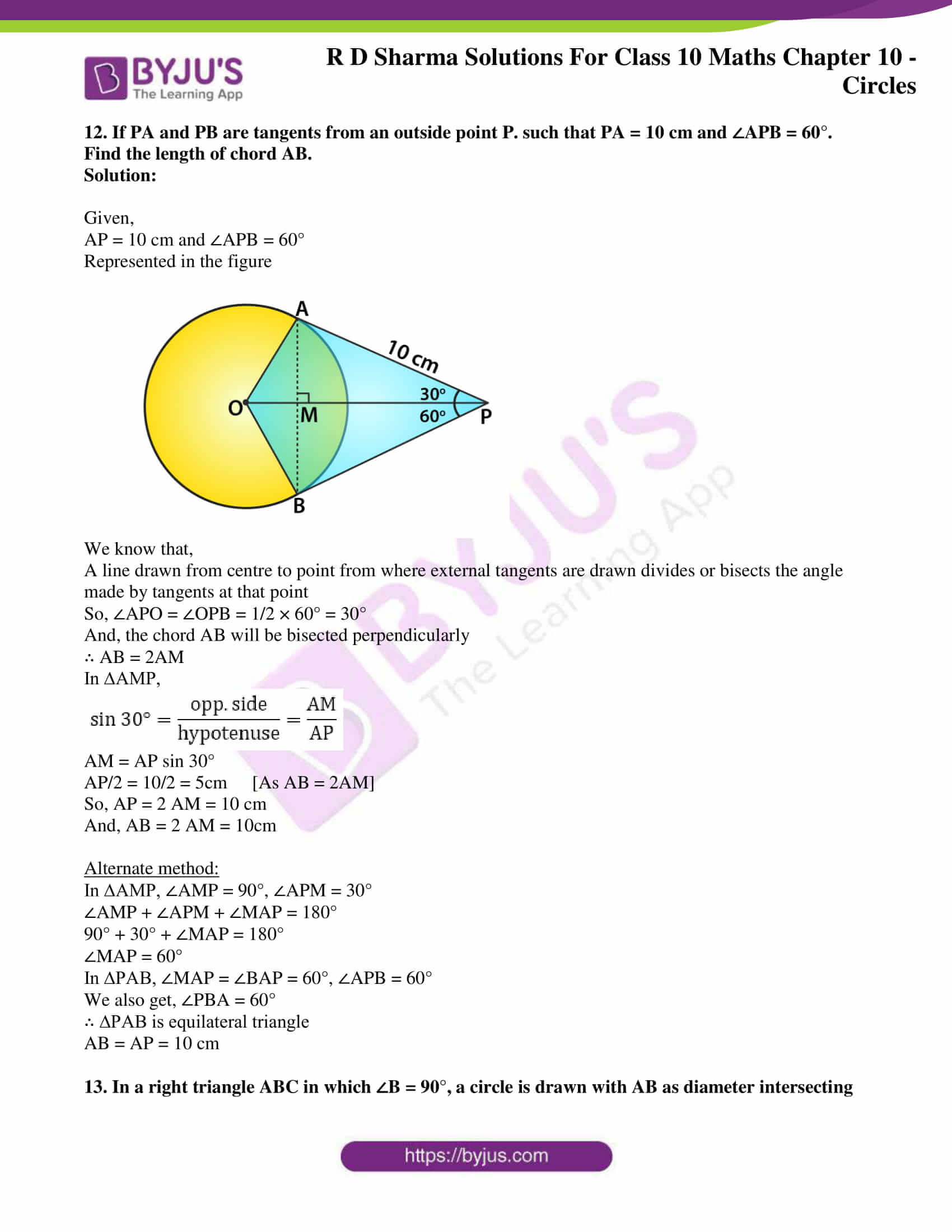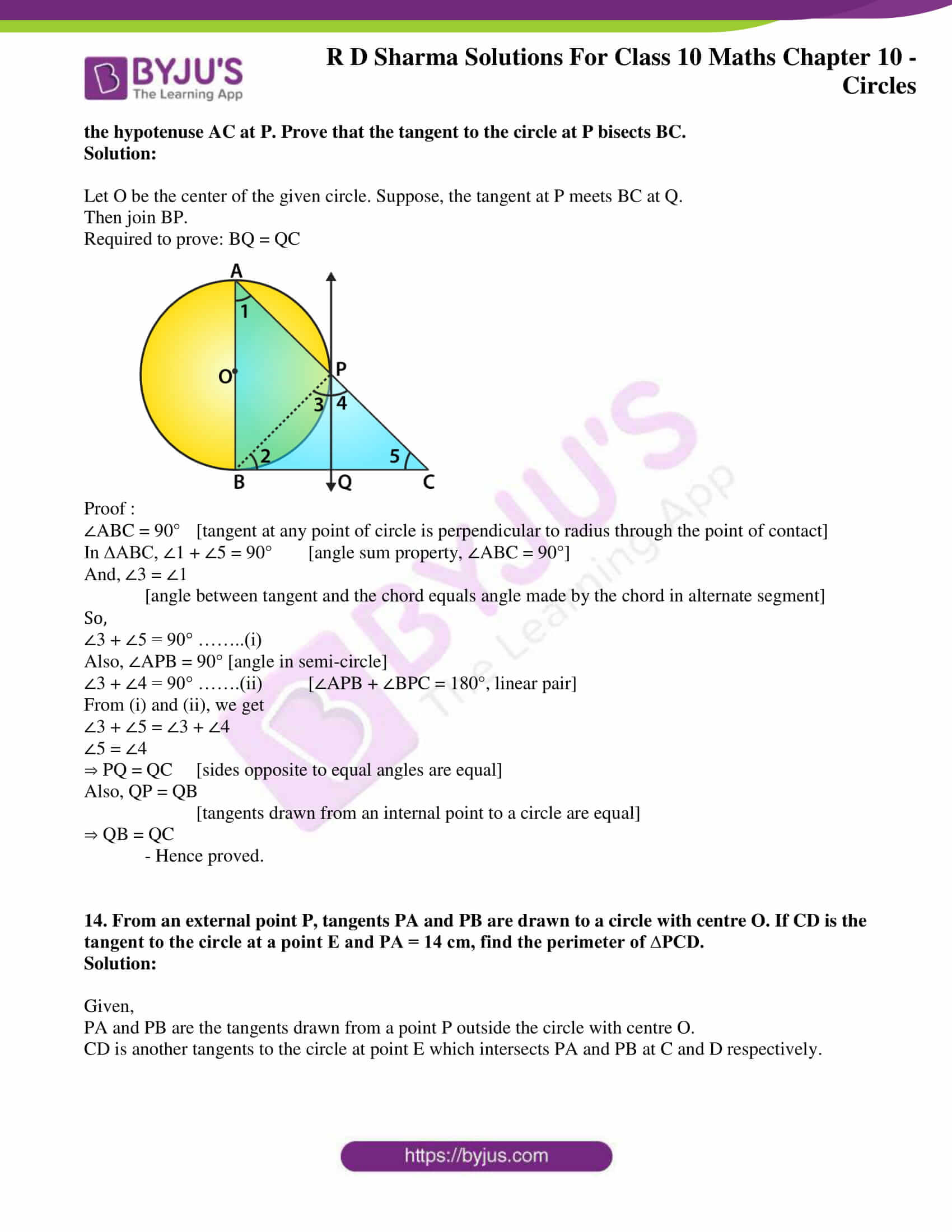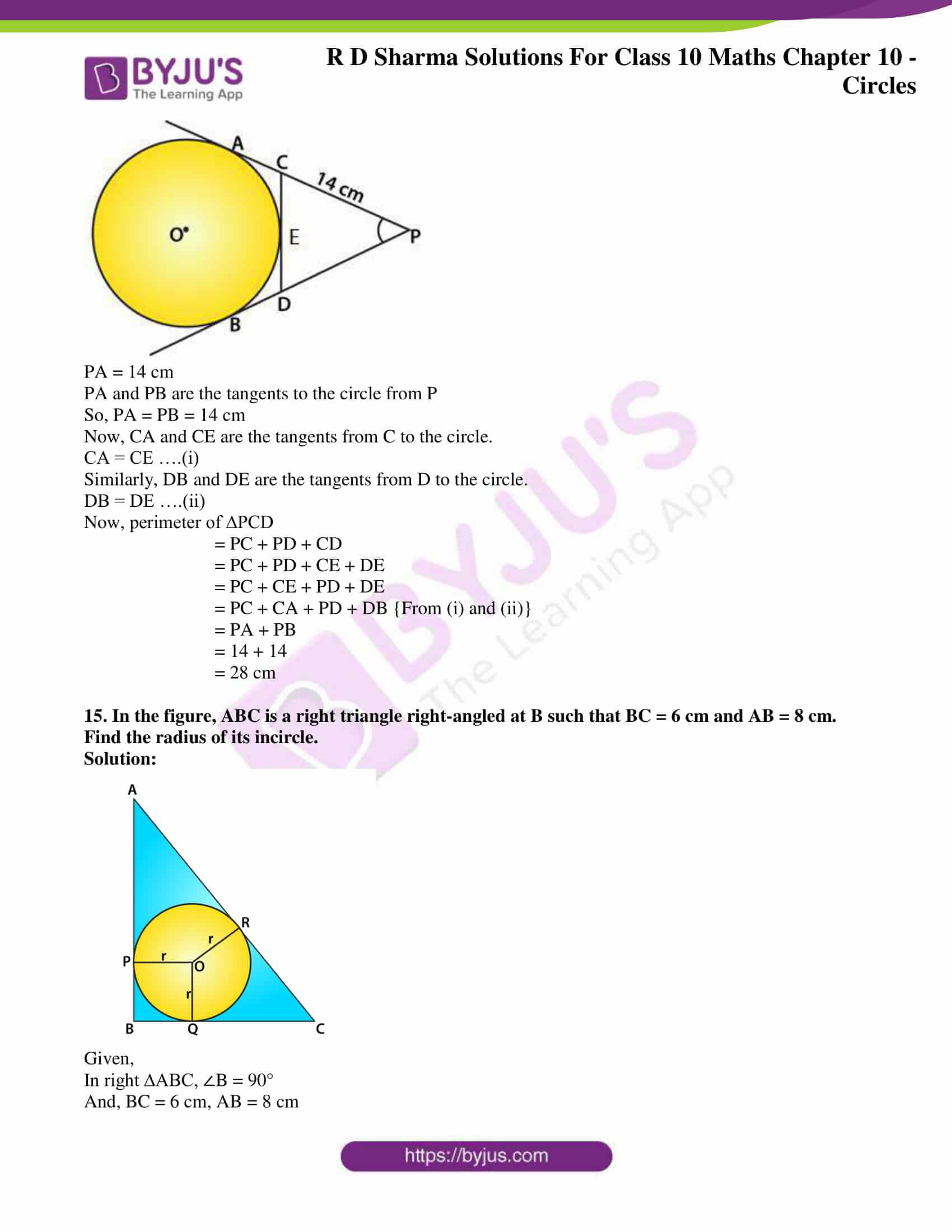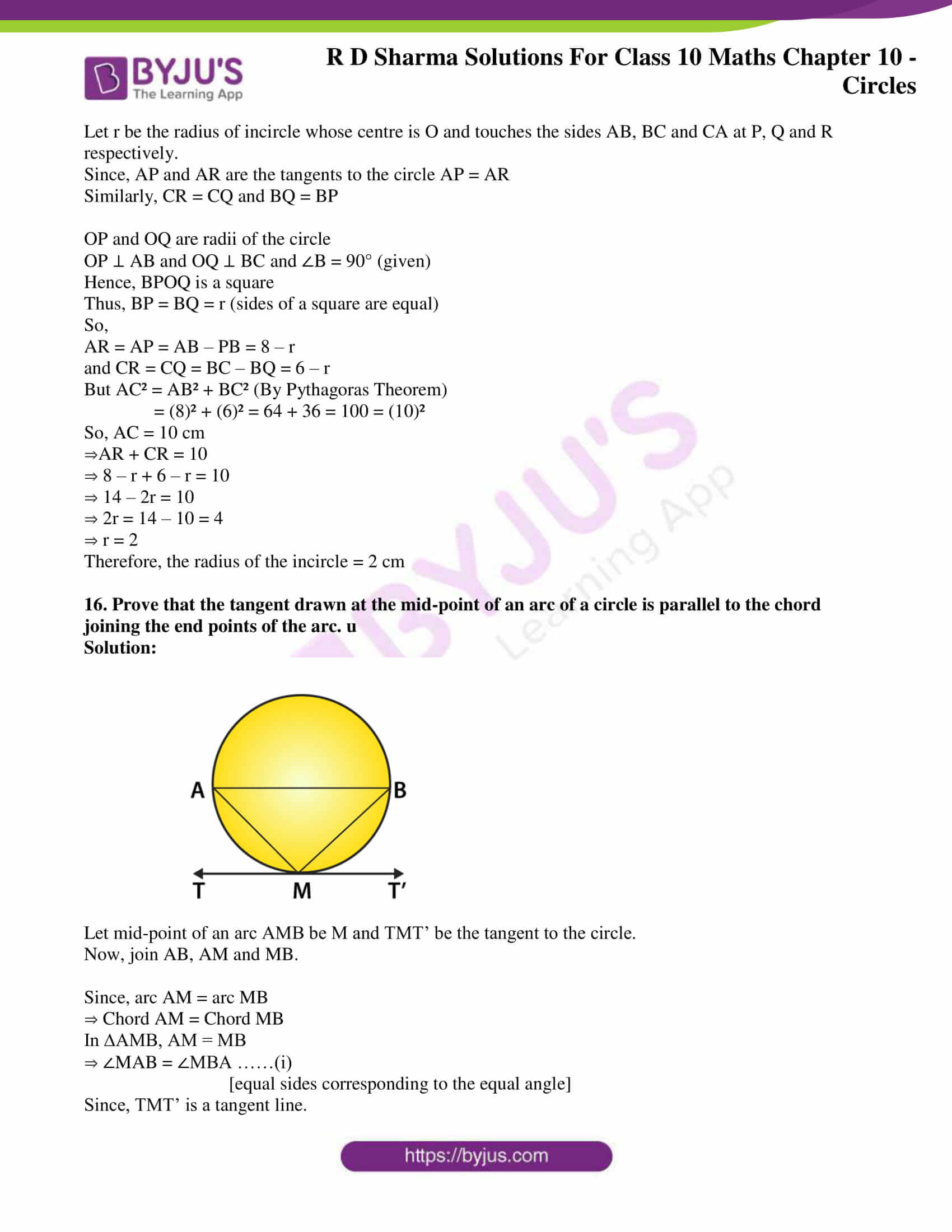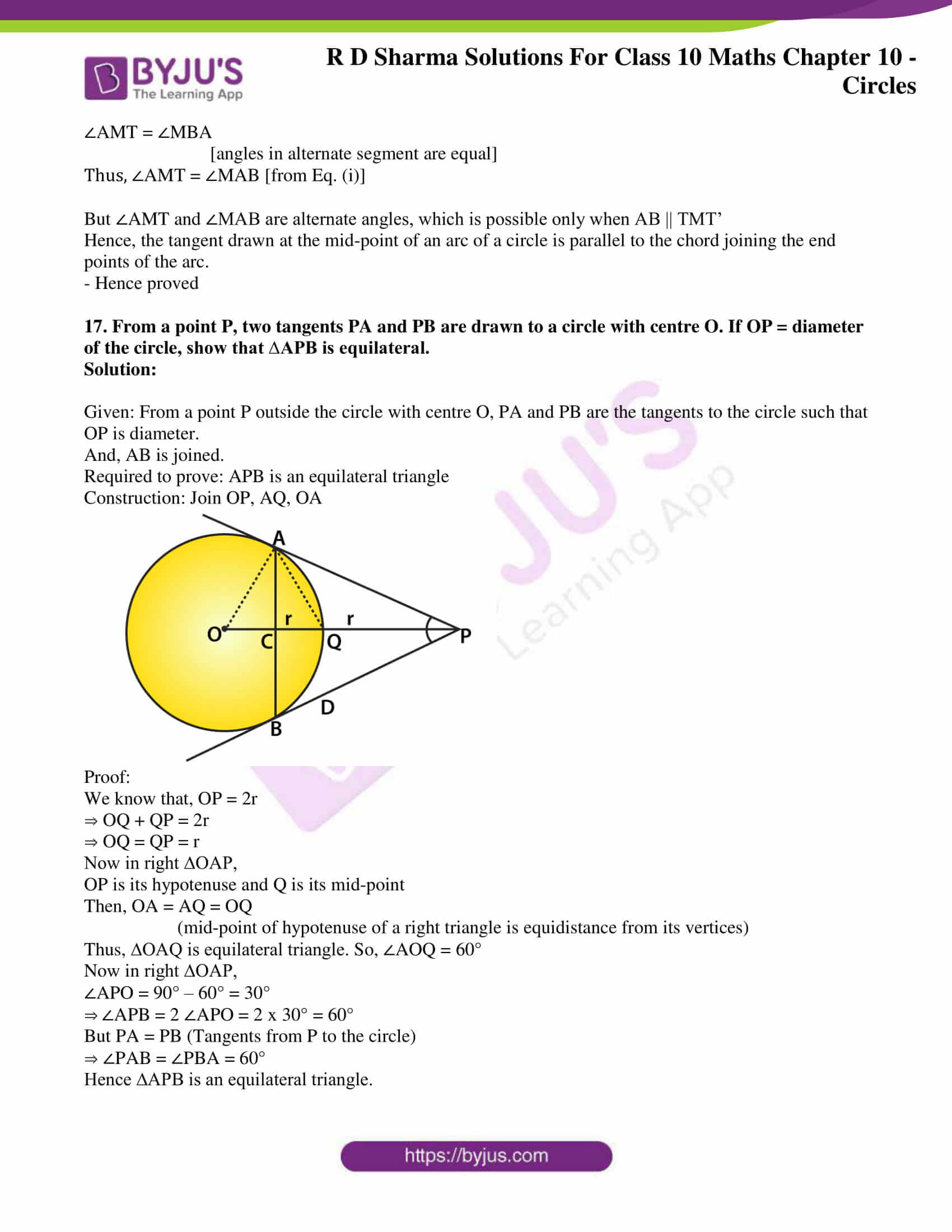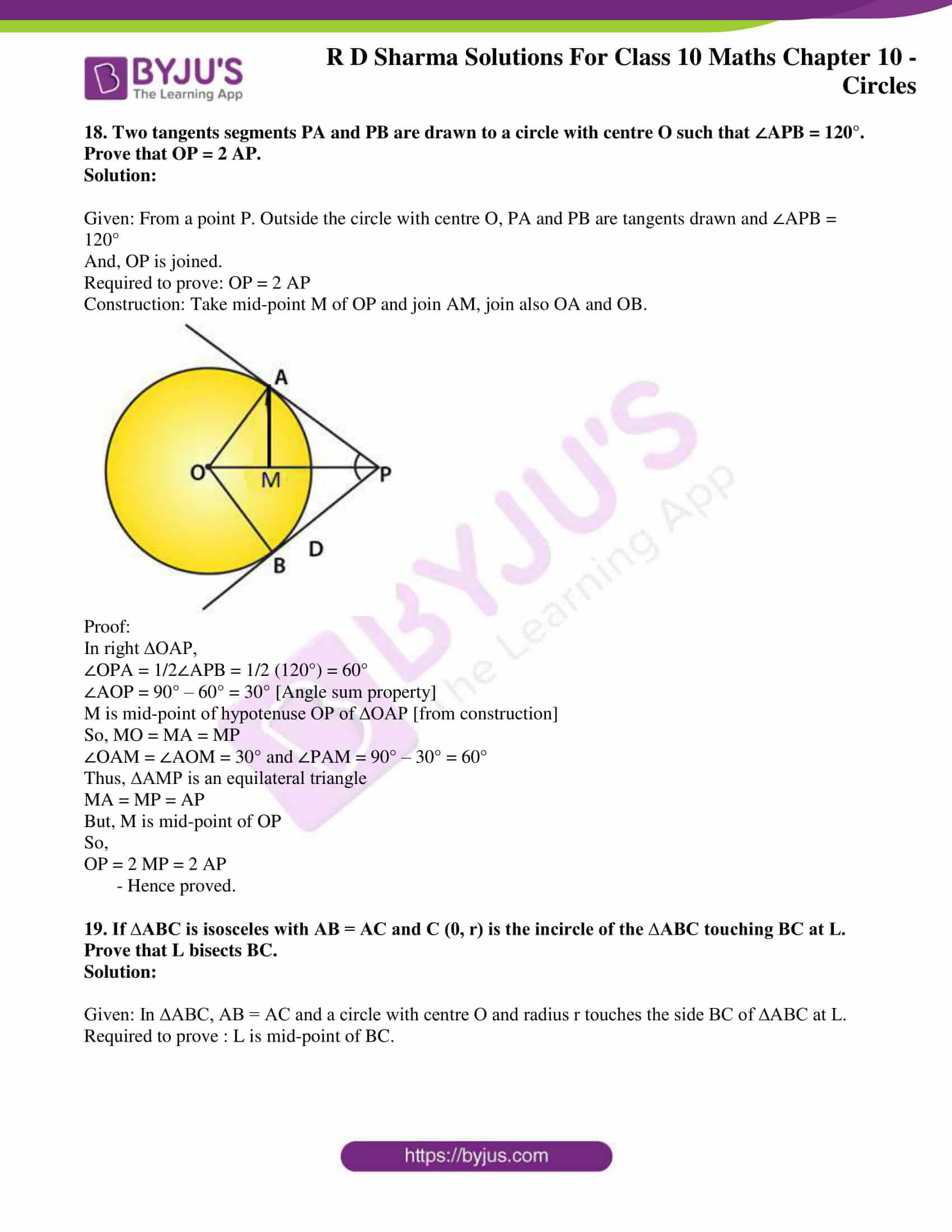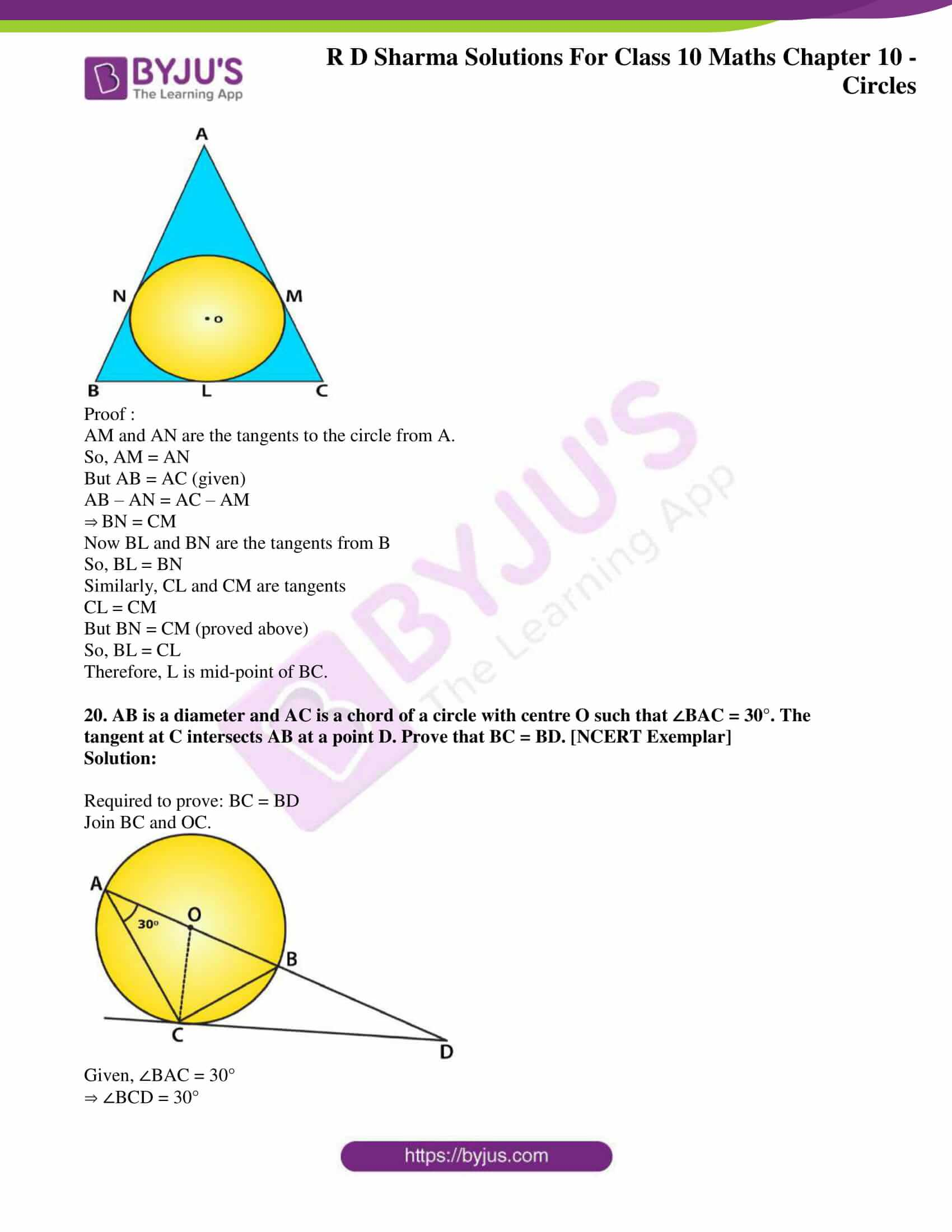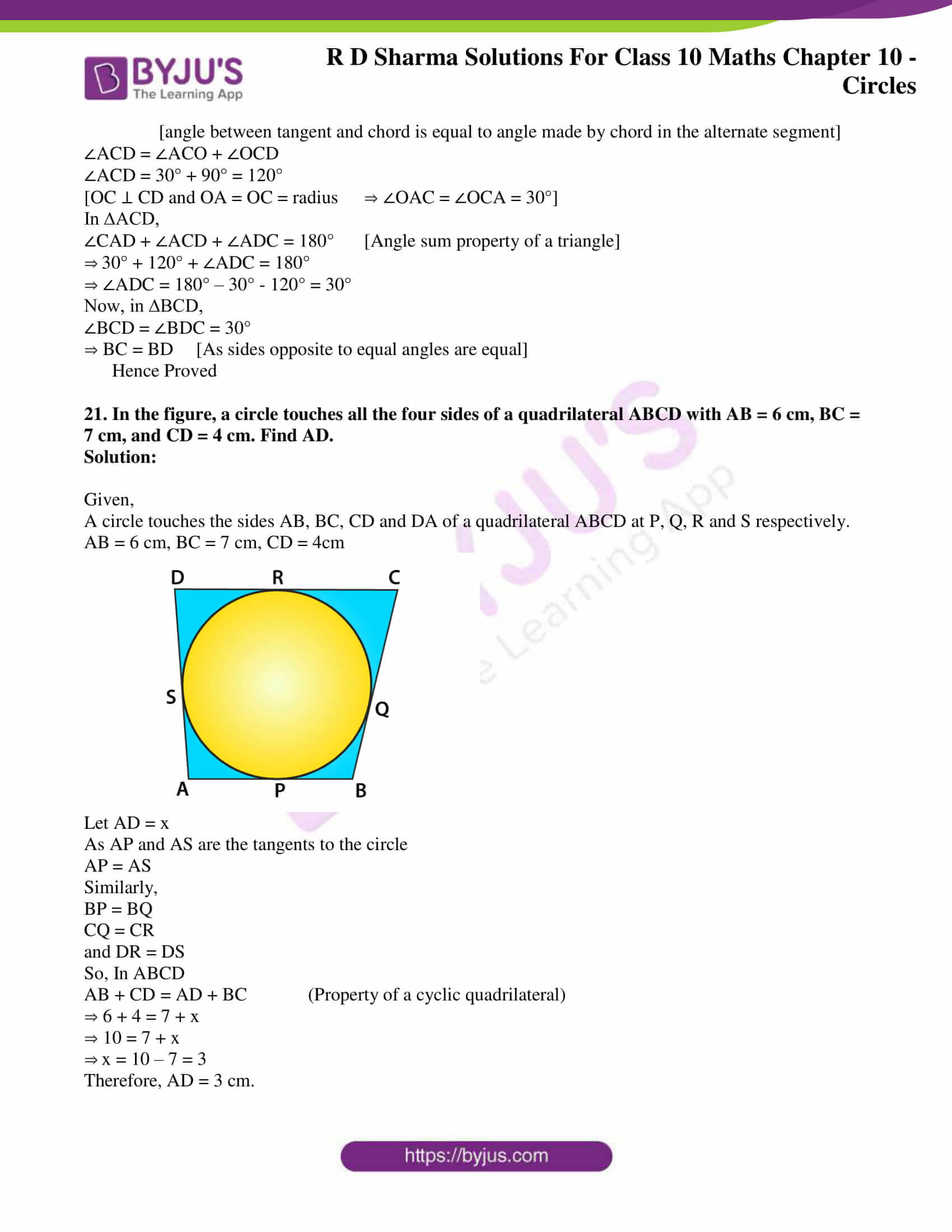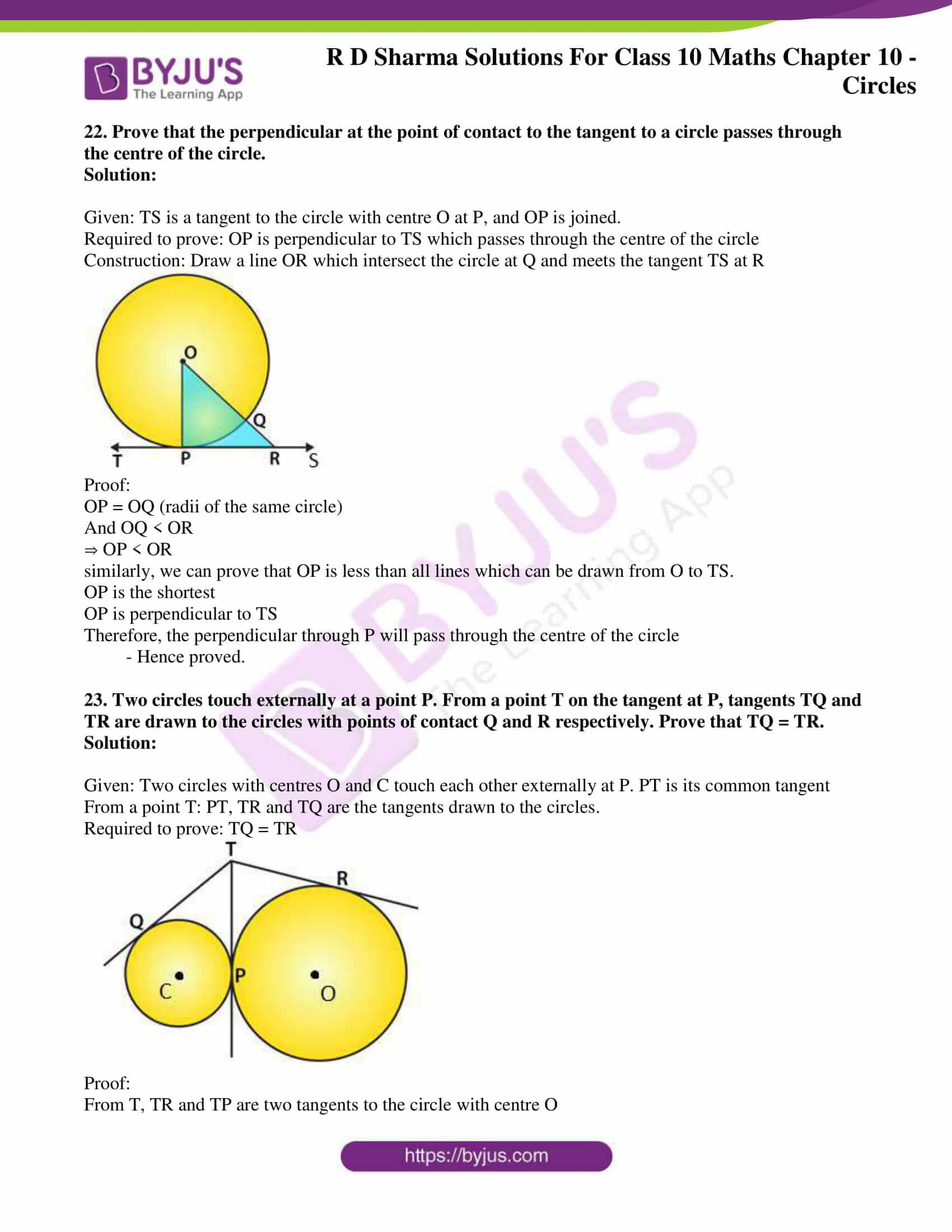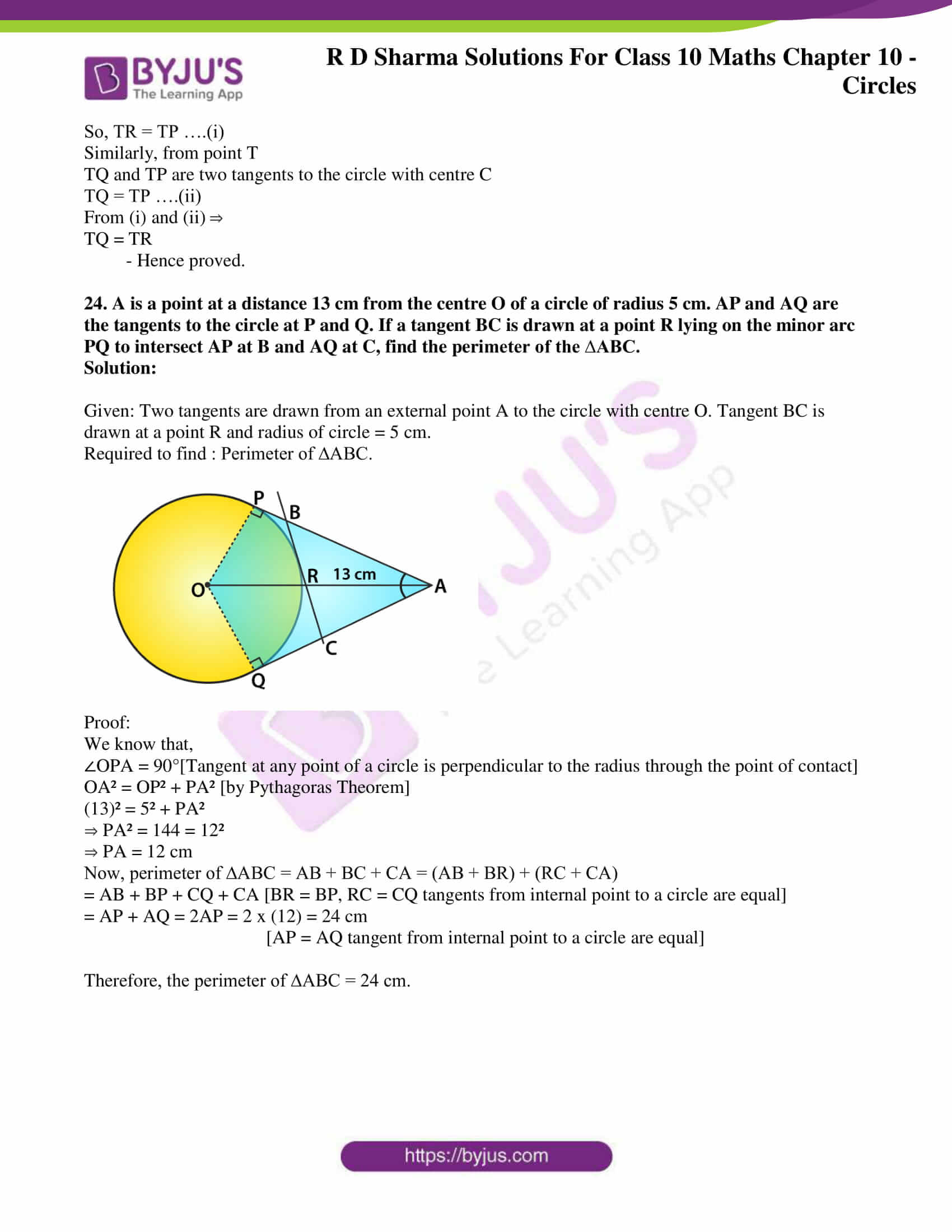### Access answers to Maths RD Sharma Solutions for Class 10 Chapter 10 Circles Exercise 10.2

1. If PT is a tangent at T to a circle whose centre is O and OP = 17 cm, OT = 8 cm. Find the length of the tangent segment PT.

Solution:

Given,

OT = radius = 8 cm

OP = 17 cm

To find: PT = length of tangent =?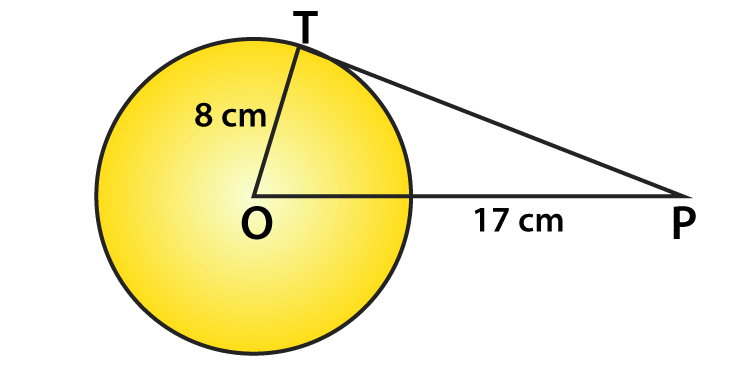Clearly, T is point of contact. And, we know that at point of contact tangent and radius are perpendicular.

∴ OTP is right angled triangle ∠OTP = 90°, from Pythagoras theorem OT2 + PT2 = OP2

82 + PT= 172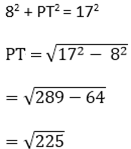∴ PT = length of tangent = 15 cm.

2. Find the length of a tangent drawn to a circle with radius 5cm, from a point 13 cm from the center of the circle.

Solution:

Consider a circle with centre O.

OP = radius = 5 cm. (given)

A tangent is drawn at point P, such that line through O intersects it at Q.

And, OQ = 13cm (given).

To find: Length of tangent PQ =?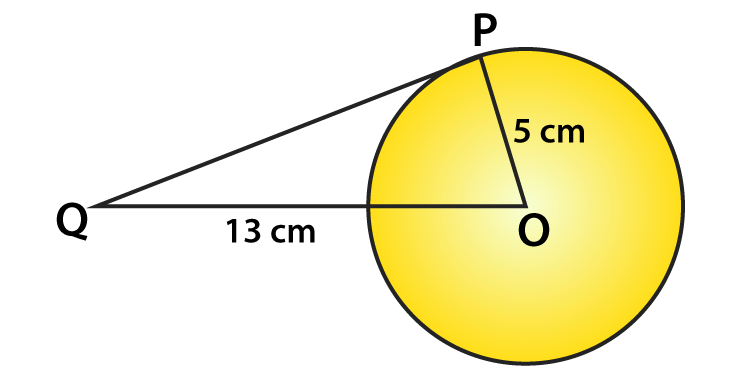We know that tangent and radius are perpendicular to each other.

∆OPQ is right angled triangle with ∠OPQ = 90°

By Pythagoras theorem we have,

OQ2 = OP2 + PQ2

⇒ 132 = 52 + PQ2

⇒ PQ2 = 169 – 25 = 144

⇒ PQ = √144

= 12 cm

Therefore, the length of tangent = 12 cm

3. A point P is 26 cm away from O of circle and the length PT of the tangent drawn from P to the circle is 10 cm. Find the radius of the circle.

Solution:

Given, OP = 26 cm

PT = length of tangent = 10 cm

To find: radius = OT = ?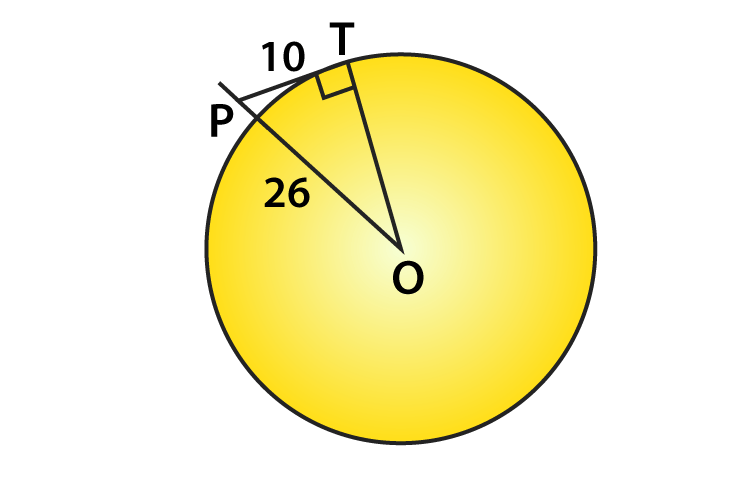We know that,

At point of contact, radius and tangent are perpendicular ∠OTP = 90°

So, ∆OTP is right angled triangle.

Then by Pythagoras theorem, we have

OP2 = OT2 + PT2

262 = OT2 + 102

OT2 = 676 – 100

OT = √576

OT = 24 cm

Thus, OT = length of tangent = 24 cm

4. If from any point on the common chord of two intersecting circles, tangents be drawn to the circles, prove that they are equal.

Solution:

Let the two circles intersect at points X and Y.

So, XY is the common chord.

Suppose ‘A’ is a point on the common chord and AM and AN be the tangents drawn from A to the circle

Then it’s required to prove that AM = AN.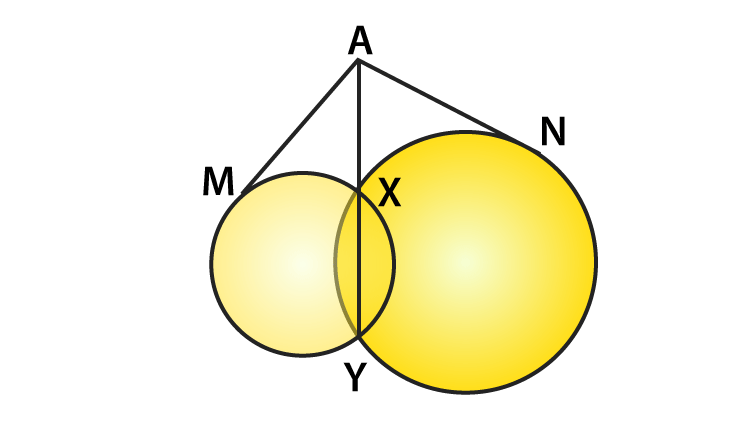In order to prove the above relation, following property has to be used.

“Let PT be a tangent to the circle from an external point P and a secant to the circle through P intersecting the circle at points A and B, then PT2 = PA × PB”

Now AM is the tangent and AXY is a secant

∴ AM2 = AX × AY … (i)

Similarly, AN is a tangent and AXY is a secant

∴ AN2 = AX × AY …. (ii)

From (i) & (ii), we have AM2 = AN2

∴ AM = AN

Therefore, tangents drawn from any point on the common chord of two intersecting circles are equal.

Hence Proved

5. If the quadrilateral sides touch the circle, prove that sum of pair of opposite sides is equal to the sum of other pair.

Solution: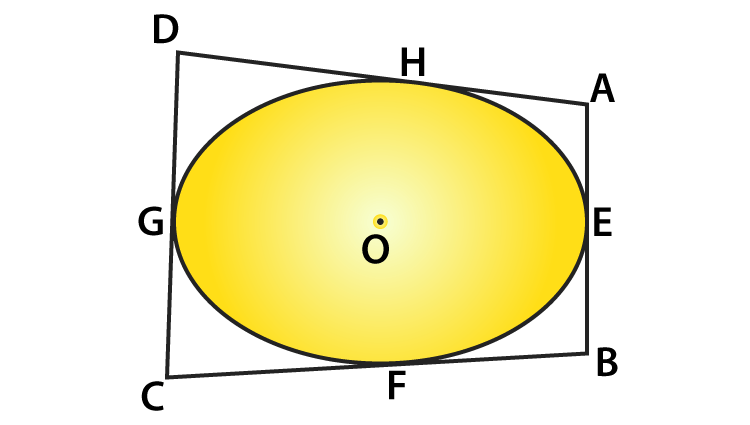Consider a quadrilateral ABCD touching circle with centre O at points E, F, G and H as shown in figure.

We know that,

The tangents drawn from same external points to the circle are equal in length.

Consider tangents:

1. From point A [AH & AE]

AH = AE … (i)

2. From point B [EB & BF]

BF = EB … (ii)

3. From point C [CF & GC]

FC = CG … (iii)

4. From point D [DG & DH]

DH = DG …. (iv)

Adding (i), (ii), (iii), & (iv)

(AH + BF + FC + DH) = [(AE + EB) + (CG + DG)]

⟹ (AH + DH) + (BF + FC) = (AE + EB) + (CG + DG)

⟹ AD + BC = AB + DC [from fig.]

Therefore, the sum of one pair of opposite sides is equal to other.

Hence Proved

6. Out of the two concentric circles, the radius of the outer circle is 5 cm and the chord AC of length 8 cm is a tangent to the inner circle. Find the radius of the inner circle.
Solution: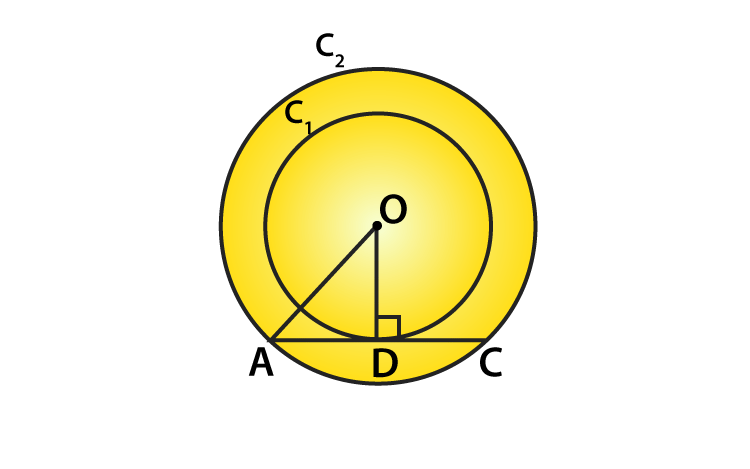Let C1 and C2 be the two circles having same center O.

And, AC is a chord which touches the C1 at point D

let’s join OD.

So, OD ⊥ AC

AD = DC = 4 cm [perpendicular line OD bisects the chord]
Thus, in right angled ∆AOD,

OA² = AD² + DO² [By Pythagoras theorem]
DO² = 5² – 4² = 25 – 16 = 9

DO = 3 cm

Therefore, the radius of the inner circle OD = 3 cm.

7. A chord PQ of a circle is parallel to the tangent drawn at a point R of the circle. Prove that R bisects the arc PRQ.
Solution: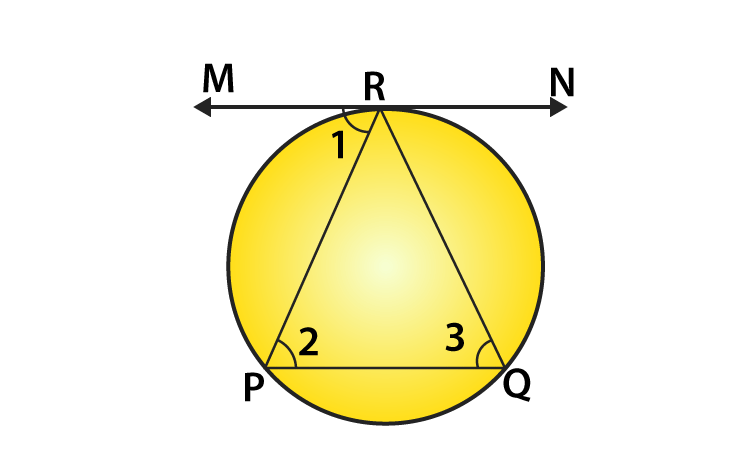Given: Chord PQ is parallel to tangent at R.

To prove: R bisects the arc PRQ.

Proof:

Since PQ || tangent at R.

∠1 = ∠2 [alternate interior angles]
∠1 = ∠3

[angle between tangent and chord is equal to angle made by chord in alternate segment]
So, ∠2 = ∠3

⇒ PR = QR [sides opposite to equal angles are equal]

Hence, clearly R bisects the arc PRQ.

8. Prove that a diameter AB of a circle bisects all those chords which are parallel to the tangent at the point A.

Solution:

Given,

AB is a diameter of the circle.

A tangent is drawn from point A.

Construction: Draw a chord CD parallel to the tangent MAN.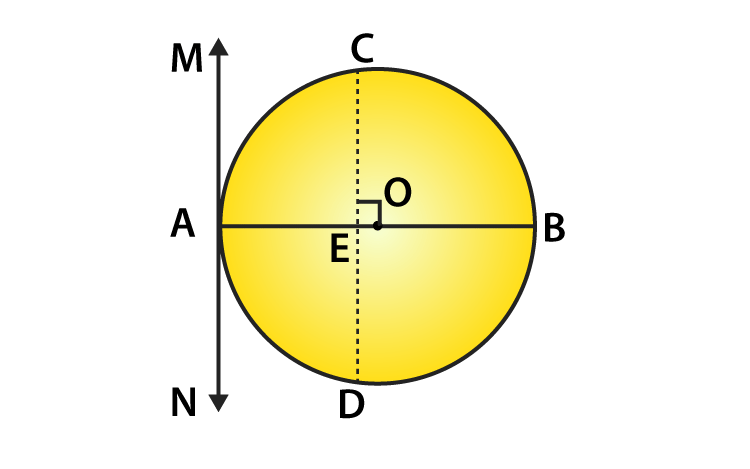So now, CD is a chord of the circle and OA is a radius of the circle.

∠MAO = 90°

[Tangent at any point of a circle is perpendicular to the radius through the point of contact]
∠CEO = ∠MAO [corresponding angles]
∠CEO = 90°

Therefore, OE bisects CD.

[perpendicular from center of circle to chord bisects the chord]
Similarly, the diameter AB bisects all the chords which are parallel to the tangent at the point A.

9. If AB, AC, PQ are the tangents in the figure, and AB = 5 cm, find the perimeter of ∆APQ.

Solution:

Given,

AB, AC, PQ are tangents

And, AB = 5 cm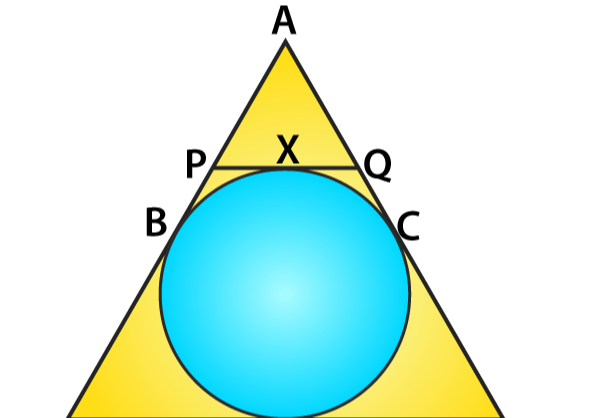Perimeter of APQ,

Perimeter = AP + AQ + PQ

= AP + AQ + (PX + QX)

We know that,

The two tangents drawn from external point to the circle are equal in length from point A,

So, AB = AC = 5 cm

From point P, PX = PB [Tangents from an external point to the circle are equal.]

From point Q, QX = QC [Tangents from an external point to the circle are equal.]

Thus,

Perimeter (P) = AP + AQ + (PB + QC)

= (AP + PB) + (AQ + QC)

= AB + AC = 5 + 5

= 10 cm.

10. Prove that the intercept of a tangent between two parallel tangents to a circle subtends a right angle at centre.

Solution:

Consider a circle with centre ‘O’ and has two parallel tangents through A & B at ends of diameter.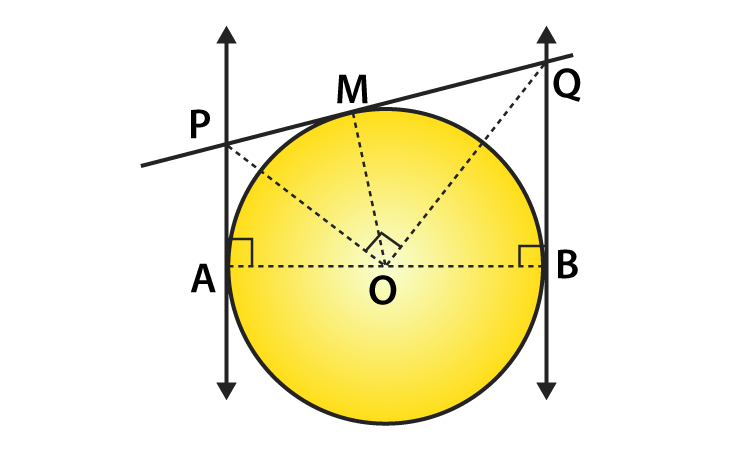Let tangent through M intersect the parallel tangents at P and Q

Then, required to prove: ∠POQ = 90°.

From fig. it is clear that ABQP is a quadrilateral

∠A + ∠B = 90° + 90° = 180° [At point of contact tangent & radius are perpendicular]

∠A + ∠B + ∠P + ∠Q = 360° [Angle sum property of a quadilateral]

So,

∠P + ∠Q = 360° – 180° = 180° … (i)

At P & Q

∠APO = ∠OPQ = 1/2 ∠P ….(ii)

∠BQO = ∠PQO = 1/2 ∠Q  ….. (iii)

Using (ii) and (iii) in (i) ⇒

2∠OPQ + 2∠PQO = 180°

∠OPQ + ∠PQO = 90° …  (iv)

In ∆OPQ,

∠OPQ + ∠PQO + ∠POQ = 180° [Angle sum property]

90° + ∠POQ = 180°           [from (iv)]

∠POQ = 180° – 90° = 90°

Hence, ∠POQ = 90°

11. In Fig below, PQ is tangent at point R of the circle with center O. If ∠TRQ = 30°, find ∠PRS.

Solution: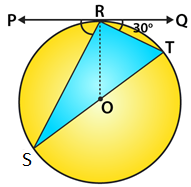Given,

∠TRQ = 30°.

At point R, OR ⊥ RQ.

So, ∠ORQ = 90°

⟹ ∠TRQ + ∠ORT = 90°

⟹ ∠ORT = 90°- 30° = 60°

It’s seen that, ST is diameter,

So, ∠SRT = 90° [ ∵ Angle in semicircle = 90°]

Then,

∠ORT + ∠SRO = 90°

∠SRO + ∠PRS = 90°

∴ ∠PRS = 90°- 30° = 60°

12. If PA and PB are tangents from an outside point P. such that PA = 10 cm and ∠APB = 60°. Find the length of chord AB.

Solution:

Given,

AP = 10 cm and ∠APB = 60°

Represented in the figure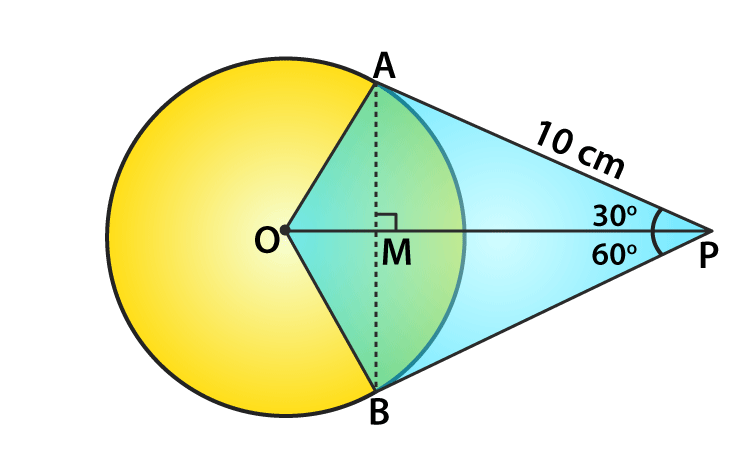We know that,

A line drawn from centre to point from where external tangents are drawn divides or bisects the angle made by tangents at that point

So, ∠APO = ∠OPB = 1/2 × 60° = 30°

And, the chord AB will be bisected perpendicularly

∴ AB = 2AM

In ∆AMP,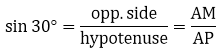AM = AP sin 30°

AP/2 = 10/2 = 5cm [As AB = 2AM]

So, AP = 2 AM = 10 cm

And, AB = 2 AM = 10cm

Alternate method:

In ∆AMP, ∠AMP = 90°, ∠APM = 30°

∠AMP + ∠APM + ∠MAP = 180°

90° + 30° + ∠MAP = 180°

∠MAP = 60°

In ∆PAB, ∠MAP = ∠BAP = 60°, ∠APB = 60°

We also get, ∠PBA = 60°

∴ ∆PAB is equilateral triangle

AB = AP = 10 cm

13. In a right triangle ABC in which ∠B = 90°, a circle is drawn with AB as diameter intersecting the hypotenuse AC at P. Prove that the tangent to the circle at P bisects BC.
Solution:

Let O be the center of the given circle. Suppose, the tangent at P meets BC at Q.

Then join BP.

Required to prove: BQ = QC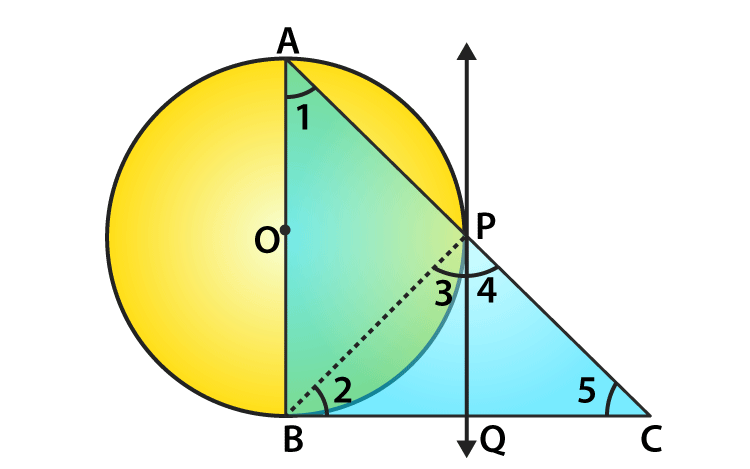Proof :

∠ABC = 90° [tangent at any point of circle is perpendicular to radius through the point of contact]
In ∆ABC, ∠1 + ∠5 = 90° [angle sum property, ∠ABC = 90°]
And, ∠3 = ∠1

[angle between tangent and the chord equals angle made by the chord in alternate segment]
So,

∠3 + ∠5 = 90° ……..(i)

Also, ∠APB = 90° [angle in semi-circle]
∠3 + ∠4 = 90° …….(ii) [∠APB + ∠BPC = 180°, linear pair]
From (i) and (ii), we get

∠3 + ∠5 = ∠3 + ∠4

∠5 = ∠4

⇒ PQ = QC [sides opposite to equal angles are equal]
Also, QP = QB

[tangents drawn from an internal point to a circle are equal]
⇒ QB = QC

– Hence proved.

14. From an external point P, tangents PA and PB are drawn to a circle with centre O. If CD is the tangent to the circle at a point E and PA = 14 cm, find the perimeter of ∆PCD.
Solution:

Given,

PA and PB are the tangents drawn from a point P outside the circle with centre O.

CD is another tangents to the circle at point E which intersects PA and PB at C and D respectively.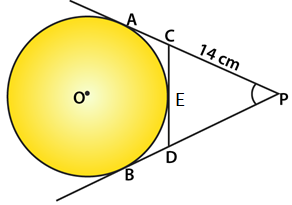PA = 14 cm

PA and PB are the tangents to the circle from P

So, PA = PB = 14 cm

Now, CA and CE are the tangents from C to the circle.

CA = CE ….(i)

Similarly, DB and DE are the tangents from D to the circle.

DB = DE ….(ii)

Now, perimeter of ∆PCD

= PC + PD + CD

= PC + PD + CE + DE

= PC + CE + PD + DE

= PC + CA + PD + DB {From (i) and (ii)}

= PA + PB

= 14 + 14

= 28 cm

15. In the figure, ABC is a right triangle right-angled at B such that BC = 6 cm and AB = 8 cm. Find the radius of its incircle.

Solution: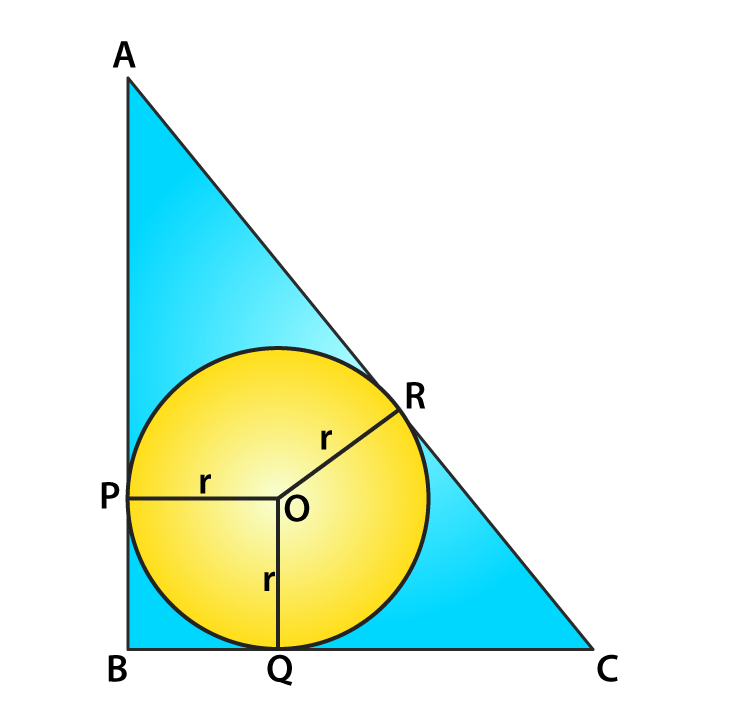Given,

In right ∆ABC, ∠B = 90°

And, BC = 6 cm, AB = 8 cm

Let r be the radius of incircle whose centre is O and touches the sides AB, BC and CA at P, Q and R respectively.

Since, AP and AR are the tangents to the circle AP = AR

Similarly, CR = CQ and BQ = BP

OP and OQ are radii of the circle

OP ⊥ AB and OQ ⊥ BC and ∠B = 90° (given)

Hence, BPOQ is a square

Thus, BP = BQ = r (sides of a square are equal)

So,

AR = AP = AB – PB = 8 – r

and CR = CQ = BC – BQ = 6 – r

But AC² = AB² + BC² (By Pythagoras Theorem)

= (8)² + (6)² = 64 + 36 = 100 = (10)²

So, AC = 10 cm

⇒AR + CR = 10

⇒ 8 – r + 6 – r = 10

⇒ 14 – 2r = 10

⇒ 2r = 14 – 10 = 4

⇒ r = 2

Therefore, the radius of the incircle = 2 cm

16. Prove that the tangent drawn at the mid-point of an arc of a circle is parallel to the chord joining the end points of the arc. u
Solution: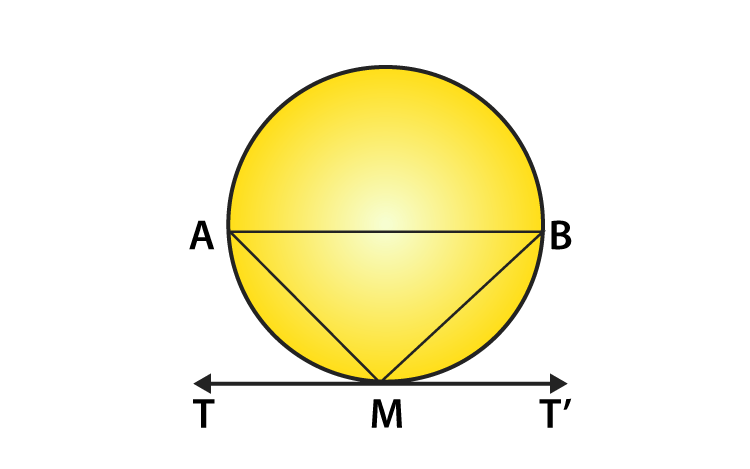Let mid-point of an arc AMB be M and TMT’ be the tangent to the circle.

Now, join AB, AM and MB.

Since, arc AM = arc MB

⇒ Chord AM = Chord MB

In ∆AMB, AM = MB

⇒ ∠MAB = ∠MBA ……(i)

[equal sides corresponding to the equal angle]
Since, TMT’ is a tangent line.

∠AMT = ∠MBA

[angles in alternate segment are equal]
Thus, ∠AMT = ∠MAB [from Eq. (i)]

But ∠AMT and ∠MAB are alternate angles, which is possible only when AB || TMT’

Hence, the tangent drawn at the mid-point of an arc of a circle is parallel to the chord joining the end points of the arc.

– Hence proved

17. From a point P, two tangents PA and PB are drawn to a circle with centre O. If OP = diameter of the circle, show that ∆APB is equilateral.
Solution:

Given: From a point P outside the circle with centre O, PA and PB are the tangents to the circle such that OP is diameter.

And, AB is joined.

Required to prove: APB is an equilateral triangle

Construction: Join OP, AQ, OA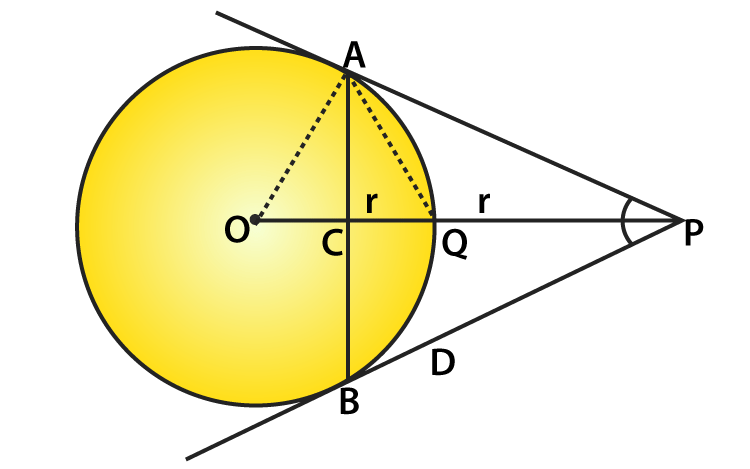Proof:

We know that, OP = 2r

⇒ OQ + QP = 2r

⇒ OQ = QP = r

Now in right ∆OAP,

OP is its hypotenuse and Q is its mid-point

Then, OA = AQ = OQ

(mid-point of hypotenuse of a right triangle is equidistance from its vertices)

Thus, ∆OAQ is equilateral triangle. So, ∠AOQ = 60°

Now in right ∆OAP,

∠APO = 90° – 60° = 30°

⇒ ∠APB = 2 ∠APO = 2 x 30° = 60°

But PA = PB (Tangents from P to the circle)

⇒ ∠PAB = ∠PBA = 60°

Hence ∆APB is an equilateral triangle.

18. Two tangents segments PA and PB are drawn to a circle with centre O such that ∠APB = 120°. Prove that OP = 2 AP.
Solution:

Given: From a point P. Outside the circle with centre O, PA and PB are tangents drawn and ∠APB = 120°

And, OP is joined.

Required to prove: OP = 2 AP

Construction: Take mid-point M of OP and join AM, join also OA and OB.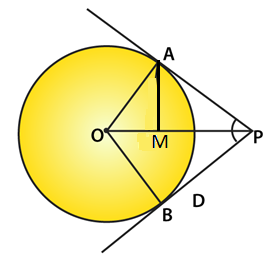Proof:

In right ∆OAP,

∠OPA = 1/2∠APB = 1/2 (120°) = 60°

∠AOP = 90° – 60° = 30° [Angle sum property]
M is mid-point of hypotenuse OP of ∆OAP [from construction]
So, MO = MA = MP

∠OAM = ∠AOM = 30° and ∠PAM = 90° – 30° = 60°

Thus, ∆AMP is an equilateral triangle

MA = MP = AP

But, M is mid-point of OP

So,

OP = 2 MP = 2 AP

– Hence proved.

19. If ∆ABC is isosceles with AB = AC and C (0, r) is the incircle of the ∆ABC touching BC at L. Prove that L bisects BC.
Solution:

Given: In ∆ABC, AB = AC and a circle with centre O and radius r touches the side BC of ∆ABC at L.

Required to prove : L is mid-point of BC.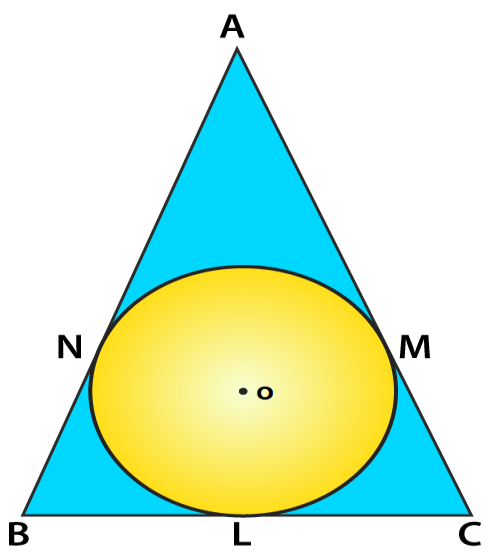Proof :

AM and AN are the tangents to the circle from A.

So, AM = AN

But AB = AC (given)

AB – AN = AC – AM

⇒ BN = CM

Now BL and BN are the tangents from B

So, BL = BN

Similarly, CL and CM are tangents

CL = CM

But BN = CM (proved above)

So, BL = CL

Therefore, L is mid-point of BC.

20. AB is a diameter and AC is a chord of a circle with centre O such that ∠BAC = 30°. The tangent at C intersects AB at a point D. Prove that BC = BD. [NCERT Exemplar]Solution:

Required to prove: BC = BD

Join BC and OC.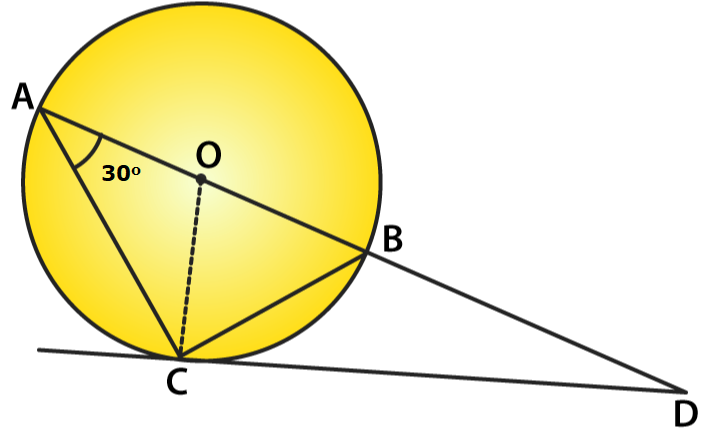Given, ∠BAC = 30°

⇒ ∠BCD = 30°

[angle between tangent and chord is equal to angle made by chord in the alternate segment]
∠ACD = ∠ACO + ∠OCD

∠ACD = 30° + 90° = 120°

[OC ⊥ CD and OA = OC = radius ⇒ ∠OAC = ∠OCA = 30°]
In ∆ACD,

∠CAD + ∠ACD + ∠ADC = 180° [Angle sum property of a triangle]
⇒ 30° + 120° + ∠ADC = 180°

⇒ ∠ADC = 180° – 30° – 120° = 30°

Now, in ∆BCD,

∠BCD = ∠BDC = 30°

⇒ BC = BD [As sides opposite to equal angles are equal]

Hence Proved

21. In the figure, a circle touches all the four sides of a quadrilateral ABCD with AB = 6 cm, BC = 7 cm, and CD = 4 cm. Find AD.
Solution:

Given,

A circle touches the sides AB, BC, CD and DA of a quadrilateral ABCD at P, Q, R and S respectively.

AB = 6 cm, BC = 7 cm, CD = 4cm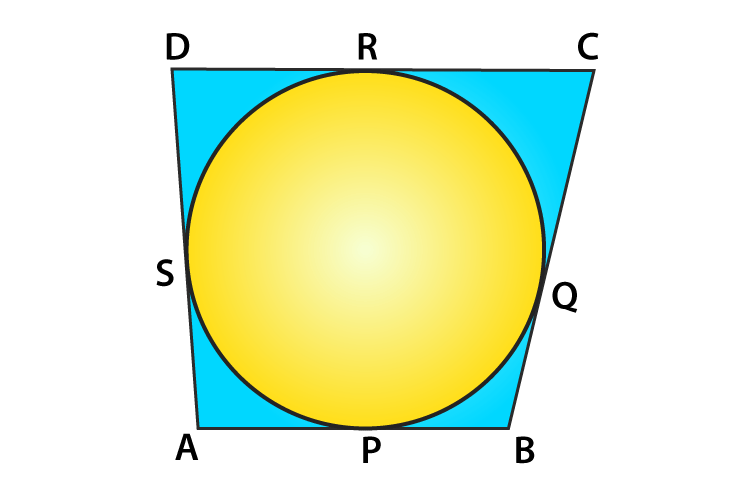Let AD = x

As AP and AS are the tangents to the circle

AP = AS

Similarly,

BP = BQ

CQ = CR

and DR = DS

So, In ABCD

AB + CD = AD + BC (Property of a cyclic quadrilateral)

⇒ 6 + 4 = 7 + x

⇒ 10 = 7 + x

⇒ x = 10 – 7 = 3

Therefore, AD = 3 cm.

22. Prove that the perpendicular at the point of contact to the tangent to a circle passes through the centre of the circle.

Solution:

Given: TS is a tangent to the circle with centre O at P, and OP is joined.

Required to prove: OP is perpendicular to TS which passes through the centre of the circle

Construction: Draw a line OR which intersect the circle at Q and meets the tangent TS at R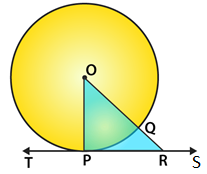Proof:

OP = OQ (radii of the same circle)

And OQ < OR

⇒ OP < OR

similarly, we can prove that OP is less than all lines which can be drawn from O to TS.

OP is the shortest

OP is perpendicular to TS

Therefore, the perpendicular through P will pass through the centre of the circle

– Hence proved.

23. Two circles touch externally at a point P. From a point T on the tangent at P, tangents TQ and TR are drawn to the circles with points of contact Q and R respectively. Prove that TQ = TR.

Solution:

Given: Two circles with centres O and C touch each other externally at P. PT is its common tangent

From a point T: PT, TR and TQ are the tangents drawn to the circles.

Required to prove: TQ = TR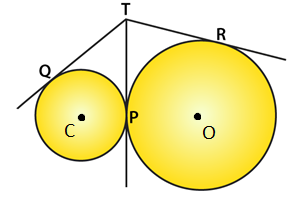Proof:

From T, TR and TP are two tangents to the circle with centre O

So, TR = TP ….(i)

Similarly, from point T

TQ and TP are two tangents to the circle with centre C

TQ = TP ….(ii)

From (i) and (ii) ⇒

TQ = TR

– Hence proved.

24. A is a point at a distance 13 cm from the centre O of a circle of radius 5 cm. AP and AQ are the tangents to the circle at P and Q. If a tangent BC is drawn at a point R lying on the minor arc PQ to intersect AP at B and AQ at C, find the perimeter of the ∆ABC.
Solution:

Given: Two tangents are drawn from an external point A to the circle with centre O. Tangent BC is drawn at a point R and radius of circle = 5 cm.

Required to find : Perimeter of ∆ABC.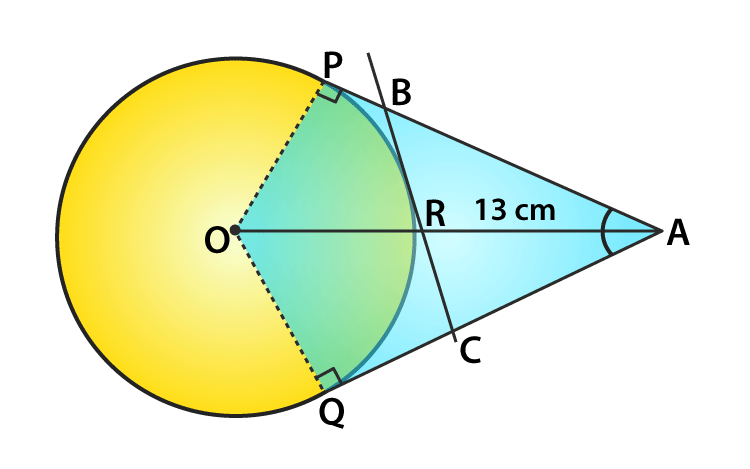Proof:

We know that,

∠OPA = 90°[Tangent at any point of a circle is perpendicular to the radius through the point of contact]
OA² = OP² + PA² [by Pythagoras Theorem]
(13)² = 5² + PA²

⇒ PA² = 144 = 12²

⇒ PA = 12 cm

Now, perimeter of ∆ABC = AB + BC + CA = (AB + BR) + (RC + CA)

= AB + BP + CQ + CA [BR = BP, RC = CQ tangents from internal point to a circle are equal]
= AP + AQ = 2AP = 2 x (12) = 24 cm

[AP = AQ tangent from internal point to a circle are equal]

Therefore, the perimeter of ∆ABC = 24 cm.